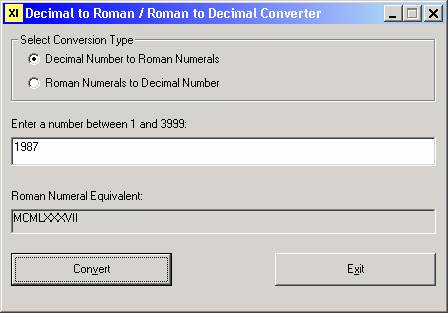PROGRAMMING EXERCISE

Roman Numerals

Write a program that will convert an integer in the range of 1 through 3999 to its equivalent in Roman numerals.

The rules for Roman numerals are as follows:

·         The basic symbols are I (= 1), V (= 5), X (= 10), L (= 50), C (= 100), D (= 500), and M (= 1000).

·         If a letter is immediately followed by one of equal or lesser value, the two numbers are added; thus, XX = 20, XV = 15, VI = 6.

·         If a letter is immediately followed by one of greater value, the first is subtracted from the second; thus IV = 4, XL = 40, CM = 900.

·         A bar over a letter multiplies it by 1000; thus, an X with a bar over it = 10,000.  Such numbers will not be addressed by this project.

Examples:

The numbers from 1 to 10 are: I, II, III, IV, V, VI, VII, VIII, IX, X.

XLVII = 47, CXVI = 116, MCXX = 1120, MCMXIV = 1914.

Note that a given symbol appears no more than three times consecutively in a number.  This is why 4 is written as "IV" instead of "IIII", and 40 is written as "XL" instead of "XXXX".

Sample runs are shown below:Enhancement

Modify the program so that it converts either way: decimal to Roman (as in the program above) OR Roman to decimal – allow the user to specify what type of conversion they want to do.

Probably the most challenging part of converting a Roman Numerals number to decimal is not the conversion itself, but rather validating the input. Basic validation would include converting keystroke input to uppercase and allowing only the characters I, V, X, L, C, D, and M to be entered. Beyond that, the following rules should be applied:

·         D, L, or V may each only appear at most one time in the string

·         M, C, X, or I may appear no more that three times consecutively in the string

·         Only I, X, and C can be used for subtraction (V, L, and D cannot). Therefore, the following pairs of letter are invalid: VX, VL, VC, VD, VM, LC, LD, LM, DM.

·         When subtracting, the value of the letter being subtracted from cannot be more than 10 times the value of letter being used for subtraction. Therefore, the following pairs of letters are invalid: IL, IC, ID, IM, XD, XM.

·         Once a letter has been used as a subtraction modifier, that letter cannot appear again in the string, unless that letter itself is subtracted from. For example, CDC is not valid (you would be subtracting 100 from 500, then adding it right back) – but CDXC (for 490) is valid. Similarly, XCX is not valid, but XCIX is.

To summarize:

C cannot follow CM or CD except in case of XC.

X cannot follow XC or XL except in the case of IX.

·         Once a letter has been subtracted from, neither it nor the next lowest multiple of 5 may appear again in the string - so neither X nor V can follow IX, neither C nor L may follow XC, and neither M nor D may follow CM.

·         A letter cannot be used as a subtraction modifier if that letter, or the next highest multiple of 5, appears previously in the string - so IV or IX cannot follow I or V, XL or XC cannot follow X or L, and CD or CM cannot follow C or D.

Sample runs are shown below. (Note: The sample program implementation uses option buttons enclosed in a frame. These controls are discussed in later tutorials.)

When the user selects "Decimal Number to Roman Numerals" for the conversion type, the program operates as in the original sample program:When the user selects "Roman Numerals to Decimal Number" for the conversion type, note that the captions change and the reverse conversion is performed:Example of invalid input:Further information on Roman Numerals can be found on these websites:

http://mathforum.org/dr.math/faq/faq.roman.html

http://www.novaroma.org/via_romana/numbers.html